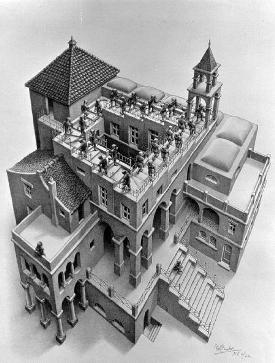Editing the Graph

Information Editing the GraphGraph, Wall, Tome Aardvark 27 January 2020 This or Better Link Drive Doc Homepage Invite All Projects

Though the original Graph aptly describes our physical knowledge, there are some minor alterations and additions to be made for it to accurately capture the current state of fundamental physics.

Eric has suggested several alterations, listed below:

• In (ii), “vector bundle X” should be changed to principal G-bundle.
• Also in (ii), “nonabelian gauge group G” should be changed to nonabelian structure group G.
• In (iii), $$R$$ and $$\tilde{R}$$ should be (complex) linear representations of G and so they are not equivalent.
• He mentioned that some info was not required, and that the Higgs is remarkably absent.

Original Graph

The Graph is a paragraph from Edward Witten's paper Physics and Geometry, at the bottom of page 20:

The Original Graph

If one wants to summarize our knowledge of physics in the briefest possible terms, there are three really fundamental observations:

(i) Spacetime is a pseudo-Riemannian manifold $$M$$, endowed with a metric tensor and governed by geometrical laws.

(ii) Over $$M$$ is a vector bundle $$X$$ with a non-abelian gauge group $$G$$.

(iii) Fermions are sections of $$(\hat{S}_{+} \otimes V_{R}) \oplus (\hat{S}_{-} \otimes V_{\tilde{R}})$$. $$R$$ and $$\tilde{R}$$ are not isomorphic; their failure to be isomorphic explains why the light fermions are light and presumably has its origins in representation difference $$\Delta$$ in some underlying theory.

All of this must be supplemented with the understanding that the geometrical laws obeyed by the metric tensor, the gauge fields, and the fermions are to be interpreted in quantum mechanical terms.

Edited Graphs

Below are modified versions of the Graph:

Edited Graph Version 2

If one wants to summarize our knowledge of physics in the briefest possible terms, there are four really fundamental observations:

1. Space-time is a pseudo-Riemannian manifold M, endowed with a metric tensor and governed by geometrical laws

2. Over M is a principal G-bundle P_{G} with a nonabelian structure group G.

3. Fermions are sections of (\hat{S}_+ \otimes V_R) \oplus (\hat{S}_- \otimes V_{\tilde{R}}). R and \tilde{R} are complex linear representations of G and thus are not isomorphic. Their failure to be isomorphic explains why the light fermions are light.

4. Yukawa couplings between the fermion field and the Higgs field endow fermions with the property of mass. Massive bosons also acquire their mass through this Higgs mechanism.

All of this must be supplemented with the understanding that the geometrical laws obeyed by the metric tensor, the gauge fields, and the fermions are to be interpreted in quantum mechanical terms.

Edited Graph Version 1

If one wants to summarise our knowledge of physics in the briefest possible terms, there are three really fundamental observations:

1. Spacetime is a pseudo-Riemannian manifold $$M$$, endowed with a metric tensor and governed by geometrical laws.
2. Over $$M$$ is a principal bundle $$P_{G}$$, with a non-abelian structure group $$G$$.
3. Fermions are sections of $$(\hat{S}_{+} \otimes V_{R}) \oplus (\hat{S}\_ \otimes V_{\bar{R}})$$. $$R$$ and $$\bar{R}$$ are not isomorphic; their failure to be isomorphic explains why the light fermions are light.
4. The masses of elementary particles are generated through the Higgs mechanism.

All of this must be supplemented with the understanding that the geometrical laws obeyed by the metric tensor, the gauge fields, and the fermions are to be interpreted in quantum mechanical terms.

Further Resources

• Eric Weinstein tweeted about the paragraph here.